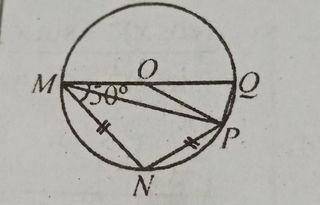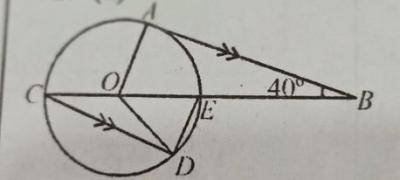### Mathematics Past Questions

4291(a) In the diagram, MNPQ is a circle with centre O, |MN| = |NP| and < OMN =  50°. Find:

(I) < MNP

(ii) < POQ

(b) Find the equation of the line which has the same gradient as 8y + 4xy = 24 and passes through the point (-8, 12)

WAEC 2020
4292(a) In the diagram, AB is a tangent to the circle with centre O, and COB is a straight line. If CD//AB and < ABE = 40°, find: < ODE.

(b) ABCD is a parallelogram in which |$$\overline{CD}$$| = 7 cm, I$$\overline{AD}$$I = 5 cm and < ADC= 125°.

(i) Illustrate the information in a diagram.

(ii) Find, correct to one decimal place, the area of the parallelogram.

(c) If x = $$\frac{1}{2}$$(1 - $$\sqrt{2}$$). Evaluate (2x$$^2$$ - 2x).

WAEC 2020
4293

Solve the following equation: $$\frac{2}{(2r - 1)}$$ - $$\frac{5}{3}$$ =  $$\frac{1}{(r + 2)}$$

• A. ( -1,$$\frac{5}{2}$$ )
• B. ( 1, - $$\frac{5}{2}$$ )
• C. ( $$\frac{5}{2}$$, 1 )
• D. (2,1)
JAMB 2021
4294

In how many ways can 2 students be selected from a group of 5 students in a debating competition?

• A. 25 ways
• B. 10 ways
• C. 15 ways
• D. 20 ways
JAMB 2021
4295

What is the rate of change of the volume V of a hemisphere with respect to its radius r when r = 2?

• A.
• B. 16π
• C.
• D.
JAMB 2021